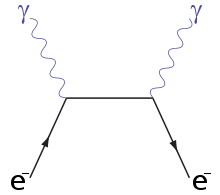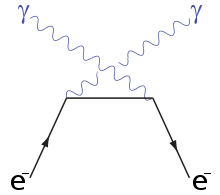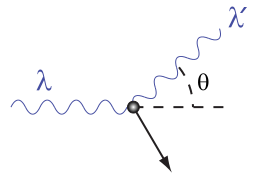# Compton scattering

 Compton scattering Feynman diagrams s-channelu-channelLight-matter interaction Low energy phenomena Photoelectric effect Mid-energy phenomena Compton scattering High energy phenomena Pair production

In physics, Compton scattering or the Compton effect is the decrease in energy (increase in wavelength) of an X-ray or gamma ray photon when it interacts with matter. Likewise, inverse Compton scattering is the phenomenon in which the photon gains energy (decreases in wavelength) upon interaction with matter. The amount the wavelength changes by is called the Compton shift. The Compton effect was observed by Arthur Holly Compton in 1923 and further verified by his graduate student Y. H. Woo during subsequent years. Arthur Compton earned the 1927 Nobel Prize in Physics for this discovery.

Compton scattering is of prime importance in radiation therapy. In material physics, it can be used to probe the wave function of the electrons in matter. It is also an important effect in gamma spectroscopy, which gives rise to the Compton edge. Compton suppression is used to detect stray scatter gamma rays to counteract this effect.

Inverse Compton scattering is important in astrophysics. The accretion disk around a black hole is thought to produce a thermal spectrum. Lower-energy photons produced from this spectrum are scattered to higher energies by relativistic electrons in the surrounding corona.

## Some significant features

Although nuclear Compton scattering exists, Compton scattering usually refers to the interaction involving only the electrons of an atom.

The Compton scattering effect is important because it demonstrates that light cannot be explained purely as a wave phenomenon. Thomson scattering, the classical theory of an electromagnetic wave scattered by charged particles, cannot explain any shift in wavelength. Light must behave as if it consists of particles in order to explain Compton scattering. Compton's experiment convinced physicists that light can behave as a stream of particles whose energy is proportional to the frequency.

The interaction between electrons and high energy photons (on the order of kiloelectron volts (keV)) results in the electron being given part of the energy (making it recoil), and a photon containing the remaining energy being emitted in a different direction from the original, such that the overall momentum of the system is conserved. If the photon still has enough energy left, the process may be repeated. In this scenario, the electron is treated as free or loosely bound. Experimental verification of momentum conservation in individual Compton scattering processes by Bothe and Geiger as well as by Compton and Simon has been important in falsifying the BKS theory.

If the photon is of lower energy, but still has sufficient energy (in general a few electronvolts (eV), right around the energy of visible light), it can eject an electron from its host atom entirely—a process known as the photoelectric effect—instead of undergoing Compton scattering. Higher energy photons (on the order of megaelectronvolts (MeV)) may be able to bombard the nucleus and cause an electron and a positron to be formed, a process called pair production.

## Applications

### Compton scattering

Compton scattering is of prime importance to radiobiology, as it happens to be the most probable interaction of high energy X rays with atomic nuclei in living beings and is applied in radiation therapy.

In material physics, Compton scattering can be used to probe the wave function of the electrons in matter in the momentum representation.

Compton scattering is an important effect in gamma spectroscopy which gives rise to the Compton edge, as it is possible for the gamma rays to scatter out of the detectors used. Compton suppression is used to detect stray scatter gamma rays to counteract this effect.

### Inverse Compton scattering

Inverse Compton scattering is important in astrophysics. In X-ray astronomy, the accretion disk surrounding a black hole is believed to produce a thermal spectrum. The lower energy photons produced from this spectrum are scattered to higher energies by relativistic electrons in the surrounding corona. This is believed to cause the power law component in the X-ray spectra (0.2-10 keV) of accreting black holes.

The effect is also observed when photons from the cosmic microwave background move through the hot gas surrounding a galaxy cluster. The CMB photons are scattered to higher energies by the electrons in this gas, resulting in the Sunyaev-Zel'dovich effect.

## The Compton shift formulaA photon of wavelength$\lambda \,$ comes in from the left, collides with a target at rest, and a new photon of wavelength$\lambda ' \,$ emerges at an angle$\theta \,$.

Compton used a combination of three fundamental formulas representing the various aspects of classical and modern physics, combining them to describe the quantum behavior of light.

The final result gives us the Compton scattering equation:$\lambda' - \lambda = \frac{h}{m_e c}(1-\cos{\theta})$

where$\lambda\,$ is the wavelength of the photon before scattering,$\lambda'\,$ is the wavelength of the photon after scattering,$m_e$ is the mass of the electron,$\theta\,$ is the angle by which the photon's heading changes,$h$ is Planck's constant, and$c$ is the speed of light.$\frac{h}{m_e c} = 2.43 \times 10^{-12}\,m$ is known as the Compton wavelength.

### Derivation

Begin with conservation of energy and conservation of momentum:$E_\gamma + E_e = E_{\gamma^\prime} + E_{e^\prime} \quad \quad (1) \,$$\vec p_\gamma = \vec{p}_{\gamma^\prime} + \vec{p}_{e^\prime} \quad \quad \quad \quad \quad (2) \,$
where$E_\gamma \,$ and$p_\gamma \,$ are the energy and momentum of the photon and$E_e \,$ and$p_e \,$ are the energy and momentum of the electron.

#### Solving (Part 1)

Now we fill in for the energy part:$E_{\gamma} + E_{e} = E_{\gamma'} + E_{e'}\,$$hf + mc^2 = hf' + \sqrt{(p_{e'}c)^2 + (mc^2)^2}\,$

The square of the second equation gives an equation for pe':$p_{e'}^2c^2 = (hf + mc^2-hf')^2-m^2c^4 \quad \quad \quad \quad \quad (3) \,$

#### Solving (Part 2)

Rearrange equation (2)$\vec{p}_{e'} = \vec{p}_\gamma - \vec{p}_{\gamma'} \,$

and square it to see$p_{e'}^2 = (\vec{p}_\gamma - \vec{p}_{\gamma'}) \cdot (\vec{p}_\gamma - \vec{p}_{\gamma'})$$p_{e'}^2 = p_{\gamma}^2 + p_{\gamma'}^2 - 2\vec{p_{\gamma}} \cdot \vec{p_{\gamma'}}$$p_{e'}^2 = p_\gamma^2 + p_{\gamma'}^2 - 2|p_{\gamma}||p_{\gamma'}|\cos(\theta) \,$

Energy and momentum of photons are connected by the relativistic equation$p_{\gamma}=\frac{E_{\gamma}}{c}$, so$E_{\gamma}^2=p_{\gamma}^2c^2=(h f)^2$.

Therefore, multiplying by$c^2$, we have also$p_{e'}^2c^2 = (h f)^2 + (h f')^2 - 2(hf)(h f')\cos{\theta} \quad \quad \quad (4)$

#### Putting it together

Now we have the two equations (three and four) for$p_{e'}^2c^2$, which we equate:$\left(h f\right)^2 + \left(h f'\right)^2 - 2h^2 ff'\cos{\theta} = (hf + mc^2-hf')^2 -m^2c^4 \,$

Next, multiply out the right-hand term$(hf + mc^2-hf')^2$ and cancel square terms on both sides and get:$-2h^2ff'\cos{\theta} = -2h^2ff'+2h(f-f')mc^2 .\,$

Then divide both sides by '$-2h$' to see$hff'\cos{\theta} = hff'-(f-f')mc^2 \,$$(f-f')mc^2 = hff'(1-\cos{\theta}) .\,$

After dividing both sides by$mc^2$ and$ff^\prime$ we get:$\frac{f-f^\prime}{f f^\prime} = \frac{h}{mc^2}\left(1-\cos \theta \right) . \,$

The left-hand side can be rewritten as simply$\frac{1}{f^\prime} - \frac{1}{f} = \frac{h}{mc^2}\left(1-\cos \theta \right) \,$

This is equivalent to the Compton scattering equation, but it is usually written in terms of wavelength rather than frequency. To make that switch use$f=\frac{c}{\lambda} \,$

so that finally,$\lambda'-\lambda = \frac{h}{mc}(1-\cos{\theta}) \,$